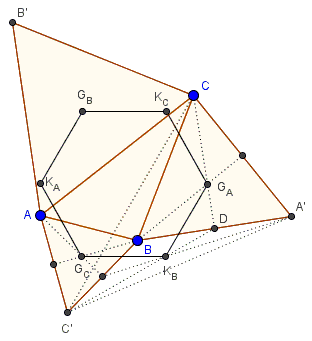# Napoleon's Hexagon

### Problem

Let $ABC',$ $BCA',$ and $CAB'$ be Napoleon's triangles constructed on the sides of $\Delta ABC.$ Define centroids $G_A,G_B,G_C,K_A,K_B,K_C$ of triangles $BCA',CAB',ABC',AB'C',A'BC',A'B'C,$ respectively.The hexagon $G_AK_CG_BK_AG_CK_B$ is regular.

### Hint

Recollect the construction and properties of Fermat's Point.

### Solution

Let $D$ be the midpoint of $A'B.$In $\Delta CC'D,$ $G_AK_B\parallel CC'$ and $G_AK_B=CC'/3.$ Similarly, for the opposite side: $G_BK_A\parallel CC'$ and $G_BK_A=CC'/3.$ It follows that $G_BK_A=G_AK_B$ and $G_BK_A\parallel G_AK_B.$ The other two pairs of opposite sides are treated in the same manner. However, Fermat's Point it is known that $AA', BB', CC'$ are all equal, meet at Fermat's point at angles of $120^{\circ},$ implying that all sides of the hexagon are equal and the angles between the adjacent sides are $120^{\circ}$ which exactly says that the hexagon is regular.

The center of the hexagon is the centroid of $\Delta ABC.$

Note now that the underlying construction of Napoleon's triangles was only important in so far as it led to the essential properties of "Fermat's lines," $AA', BB' CC'.$ As long as the three line segments are equal, at $120^{\circ}$ angles to each other, and the triangles $ABC$ and $A'B'C'$ have the same orientation, the hexagon defined by the six points $A,A',B,B',C,C'$ will remain regular. I placed the dynamic illustration on a separate page.

### Acknowledgment

The problem has been posted and proved by Dao Thanh Oai at the CutTheKnotMath facebook page.### Napoleon's Theorem